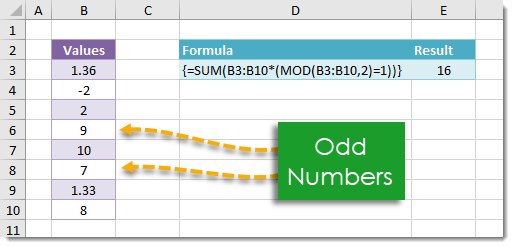## Example

{=SUM(B3:B10*(MOD(B3:B10,2)=1))}

## Generic Formula

{=SUM(Range*(MOD(Range,2)=1))}

Note: This is an array formula. Do not type out the {} brackets. Hold Ctrl + Shift then press Enter while in Edit Mode to create an array formula.

Range – This is the range of values which you would like to sum all odd numbers from.

## What It Does

This formula will sum all the odd numbers from a given range of values. An odd number is defined as any number that has a remainder of 1 when divided by 2.

## How It Works

The MOD function returns the remainder of a number on division by another number. For example MOD(7,2) will return 1 since the remainder of 7 divided by 2 is 1. We use the MOD function to test if a number is odd. A number will be odd if and only if the remainder on division by 2 equals 1. In other words, N is odd if and only if MOD(N,2)=1.

MOD(Range,2)=1 will create an array of Boolean values where TRUE will mean the number is odd and FALSE will mean the number is not odd. We then multiply this Boolean array by the Range to get an array where each value is either an odd value from the Range or a 0. This is because N*TRUE equals N and N*FALSE equals 0 for any number N.

In our example MOD({1.36;-2;2;9;10;7;1.33;8},2)=1 will result in the following Boolean array.

{FALSE;FALSE;FALSE;TRUE;FALSE;TRUE;FALSE;FALSE}

When we multiply this by Range we get {0;0;0;9;0;7;0;0}. Then SUM({0;0;0;9;0;7;0;0}) results in 16 which is the sum of all odd numbers in our range of values.